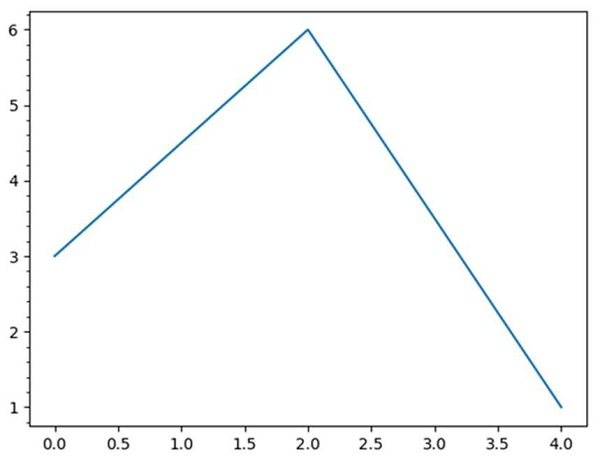# How to turn on minor ticks only on the y-axis Matplotlib?

First, we can create fig, ax using subplots() and then, we can plot the lines. After that, using ax.yaxis.set_minor_locator(tck.AutoMinorLocator()), we can turn on the minor ticks.

## Steps

• Create fig and ax variables using subplots method, where default nrows and ncols are 1.

• Plot the line using two lists.

• Set the locator of the minor ticker.

• Dynamically find minor tick positions based on the positions of major ticks. The scale must be linear with major ticks evenly spaced.

• Using plt.show() method, we can show the figure.

## Example

import matplotlib.pyplot as plt
import matplotlib.ticker as tck
fig, ax = plt.subplots()
plt.plot([0, 2, 4], [3, 6, 1])
ax.yaxis.set_minor_locator(tck.AutoMinorLocator())
plt.show()

## Output